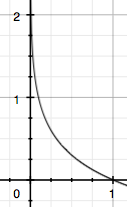# 交叉熵

1 信息量

I(x0)=−log(p(x0))2 熵

A 电脑正常开机 0.7 -log(p(A))=0.36
B 电脑无法开机 0.2 -log(p(B))=1.61
C 电脑爆炸了 0.1 -log(p(C))=2.30

H(X)=−∑i=1np(xi)log(p(xi))

H(X)=−[p(A)log(p(A))+p(B)log(p(B))+p(C))log(p(C))]=0.7×0.36+0.2×1.61+0.1×2.30=0.804

H(X)=−∑i=1np(xi)log(p(xi))=−p(x)log(p(x))−(1−p(x))log(1−p(x))
3 相对熵（KL散度）

In the context of machine learning, DKL(P‖Q) is often called the information gain achieved if P is used instead of Q.

KL散度的计算公式：
DKL(p||q)=∑i=1np(xi)log(p(xi)q(xi))(3.1)

n为事件的所有可能性。
DKLDKL的值越小，表示q分布和p分布越接近
4 交叉熵

DKL(p||q)==∑i=1np(xi)log(p(xi))−∑i=1np(xi)log(q(xi))−H(p(x))+[−∑i=1np(xi)log(q(xi))]
DKL(p||q)=∑i=1np(xi)log(p(xi))−∑i=1np(xi)log(q(xi))=−H(p(x))+[−∑i=1np(xi)log(q(xi))]

H(p,q)=−∑i=1np(xi)log(q(xi))
H(p,q)=−∑i=1np(xi)log(q(xi))

1 为什么要用交叉熵做loss函数？

loss=12m∑i=1m(yi−yi^)2
loss=12m∑i=1m(yi−yi^)2

MSE在线性回归问题中比较好用，那么在逻辑分类问题中还是如此么？

2 交叉熵在单分类问题中的使用

loss=−∑i=1nyilog(yi^)(2.1)
(2.1)loss=−∑i=1nyilog(yi^)

* 猫 青蛙 老鼠
Label 0 1 0
Pred 0.3 0.6 0.1

loss==−(0×log(0.3)+1×log(0.6)+0×log(0.1)−log(0.6)
loss=−(0×log(0.3)+1×log(0.6)+0×log(0.1)=−log(0.6)

loss=−1m∑j=1m∑i=1nyjilog(yji^)
loss=−1m∑j=1m∑i=1nyjilog(yji^)
m为当前batch的样本数

3 交叉熵在多分类问题中的使用

* 猫 青蛙 老鼠
Label 0 1 1
Pred 0.1 0.7 0.8

loss=−ylog(y^)−(1−y)log(1−y^)
loss=−ylog(y^)−(1−y)log(1−y^)

loss猫loss蛙loss鼠===−0×log(0.1)−(1−0)log(1−0.1)=−log(0.9)−1×log(0.7)−(1−1)log(1−0.7)=−log(0.7)−1×log(0.8)−(1−1)log(1−0.8)=−log(0.8)
loss猫=−0×log(0.1)−(1−0)log(1−0.1)=−log(0.9)loss蛙=−1×log(0.7)−(1−1)log(1−0.7)=−log(0.7)loss鼠=−1×log(0.8)−(1−1)log(1−0.8)=−log(0.8)

loss=∑j=1m∑i=1n−yjilog(yji^)−(1−yji)log(1−yji^)
loss=∑j=1m∑i=1n−yjilog(yji^)−(1−yji)log(1−yji^)

posted @ 2019-06-03 15:59  胡里糊涂  阅读(145)  评论(0编辑  收藏